Next: Ferromagnetism Up: Applications of Statistical Thermodynamics Previous: Maxwell Velocity Distribution

# Effusion

Consider a dilute gas enclosed in a container. Let us calculate how many molecules per unit time strike a unit area of the container wall.

Consider a wall element of area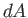. The-axis is oriented so as to point along the outward normal of this element. Let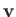represent a molecular velocity. The Cartesian components of this velocity are conveniently written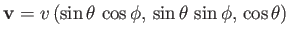, where the standard spherical angles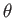and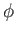specify the velocity vector's direction. Let us concentrate on those molecules, in the immediate vicinity of the wall element, whose velocities are such that they lie betweenand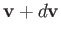. In other words, the velocities are such that their magnitudes lies between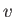and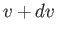, their polar angles lie betweenand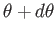, and their azimuthal angles lie betweenand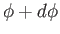. Molecules of this type suffer a displacement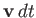in the infinitesimal time interval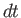. Hence, all molecules that lie within the infinitesimal cylinder of cross-sectional area, and length(whose axis subtends an anglewith the-axis, and whose two ends are parallel to the wall element), will strike the wall element within the time interval, whereas those molecules outside this cylinder will not. (See Figure 7.8.) The volume of the cylinder is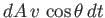, whereas the number of molecules per unit volume in the prescribed velocity range is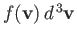. Hence, the number of molecules of this type that strike the areain the time intervalis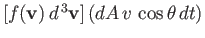. Dividing this expression by the area, and the time interval, we obtain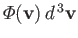, which is defined as the number of molecules, with velocities in the rangeto, that strike a unit area of the wall per unit time. Thus,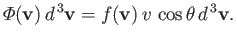(7.233)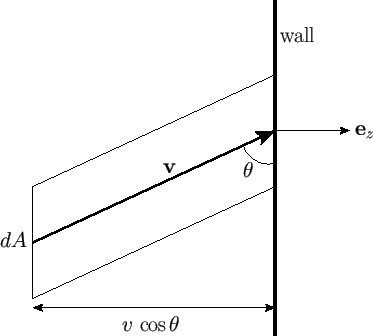Let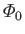be the total number of molecules that strike a unit area of the wall per unit time. This quantity is simply obtained by summing (7.233) over all possible molecular velocities that would cause molecules to collide with the element of area. This means that we have to sum over all possible speeds,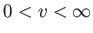, all possible azimuthal angles,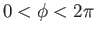, and all polar angles in the range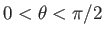. (Those molecules with polar angles in the range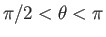have their velocity directed away from the wall, and will, hence, not collide with it.) In other words, we have to sum over all possible velocities,, subject to the restriction that the velocity component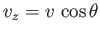is positive. Thus, we have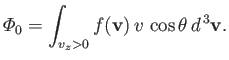(7.234)

The element of volume in velocity space can be expressed in spherical coordinates: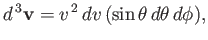(7.235)

where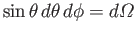is just an element of solid angle. Moreover, in thermal equilibrium,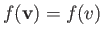is only a function of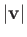. Hence, Equation (7.234) becomes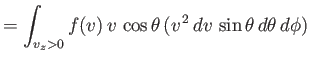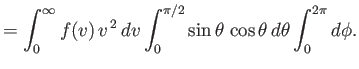(7.236)

The integral overgives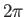, whereas that overreduces to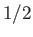. It follows that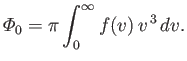(7.237)

Now, the mean molecular speed is written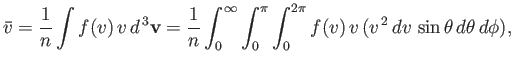(7.238)

which reduces to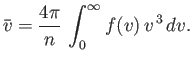(7.239)

Hence, we deduce from Equation (7.237) that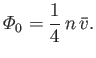(7.240)

The previous result can be combined with Equation (7.227), and the ideal equation of state,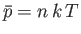, to give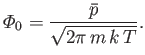(7.241)

If a sufficiently small hole is made in the wall of the container then the equilibrium of the gas inside the container is disturbed to a negligible extent. [In practice, a sufficiently small hole is one that is small compared to the molecular mean-free-path (i.e., the mean distance travelled between collisions) inside the container.] In this case, the number of molecules that emerge through the small hole is the same as the number of molecules that would strike the area occupied by the hole if the latter were closed off. The process by which molecules emerge through a small hole is called effusion. The number of molecules that have speeds in the range betweenand, and which emerge through a small hole of area, into the solid angle range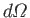, in the forward direction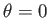, is given by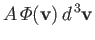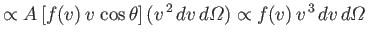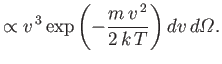(7.242)

Now, it is difficult to directly verify the Maxwell velocity distribution. However, this distribution can be verified indirectly by measuring the velocity distribution of atoms effusing from a small hole in an oven. As we have just seen, the predicted velocity distribution of the escaping atoms varies like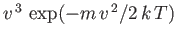, in contrast to the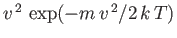variation of the velocity distribution inside the oven. There is good agreement between the experimentally measured velocity distribution of effusing atoms and the theoretical prediction.

As another example, consider a container that is divided into two parts by a partition containing a small hole. The container is filled with gas, but one part of the container is maintained at the temperature, and the other at the temperature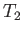. Let us calculate the relationship between the mean gas pressures,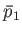and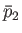, respectively, in the two parts, when the system is in equilibrium. (That is, when a situation is reached in which neitheror, nor the amount of gas in either parts, changes with time.) If the hole is larger than the molecular mean-free-path then the relationship is simply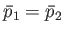--otherwise, the pressure difference would give rise to bulk motion of the gas from one side of the container to the other, until the pressures were equalized. But, if the dimensions of the hole are less than the mean-free-path then we are dealing with effusion through the hole, rather than hydrodynamical flow. In this case, the equilibrium condition requires that the mass of gas on each side remain constant. In other words, the effusion rates in both directions must be equal. Thus, it follows from Equation (7.241) that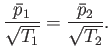(7.243)

Thus, the pressures are not equal. Instead, a higher pressure prevails in the part of the container held at a higher temperature.Next: Ferromagnetism Up: Applications of Statistical Thermodynamics Previous: Maxwell Velocity Distribution
Richard Fitzpatrick 2016-01-25